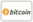Bookcover of The modified Adomian decomposition method
Booktitle:

# The modified Adomian decomposition method

## Singular initial-value problems in second and higher-order ordinary differential equations of Lane-Emden

LAP LAMBERT Academic Publishing (2012-12-29 )eligible for voucher
ISBN-13:

### 978-3-659-31479-7

ISBN-10:
365931479X
EAN:
9783659314797
Book language:
English
Blurb/Shorttext:
Adomian’s decomposition method is a useful and powerful method for solving linear and nonlinear ordinary differential equations. In this book we consider singular initial value problems, singular boundary value problems, boundary value problems and nonlinear oscillatory equations. Singular initial-value problems in second-order ordinary differential equations of Lane-Emden type are investigated.In this study, we shall introduce a further development in the Adomian decomposition method to overcome the difficulty at linear and non-linear Lane-Emden-like equations; especially when the singularity appears two times on the left -hand side of this type of equations. A more general concept of this type of initial-value problems is introduced with various examples. The numerical results of these examples are compared with exact solutions when available. A fast and accurate algorithm is developed for the solution of higher order boundary value problems with boundary conditions. A modified form of the Adomian decomposition method is applied to construct the numerical solution for such problems.
Publishing house:
Website:
https://www.lap-publishing.com/
By (author) :
Yahya Hasan
Number of pages:
112
Published at:
2012-12-29
Stock:
Available
Category:
Mathematics
Price:
49.00 €
Keywords:
boundary value problems, Singular initial value problems, Oscillatory equations### Categories